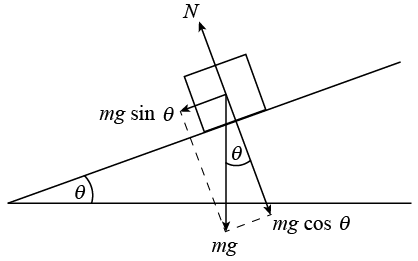# An object with a mass of 4 kg is on a plane with an incline of  - pi/4 . If it takes 7 N to start pushing the object down the plane and 6 N to keep pushing it, what are the coefficients of static and kinetic friction?

Feb 22, 2018where:

theta = pi/4 = 45°

Moreover, recall,

$\Sigma \vec{F} = m a$

Let's determine the normal force,

$\Sigma {F}_{y} = {F}_{N} - m g \cos \theta = 0$
$\implies {F}_{N} = m g \cos \theta$

and recall that to overcome the static friction a force equal to that friction must be exerted on the object.

Hence,

$\Sigma {F}_{x} = {F}_{P} + m g \sin \theta = {\mu}_{s} \cdot {F}_{N}$

$\implies {\mu}_{s} = \frac{{F}_{P} + m g \sin \theta}{m g \cos \theta} \approx 1.25$

If we wish to keep pushing the object down the incline, we need to push it with a force greater than or equal to its kinetic friction. Assuming that we are pushing the object with the least amount of force before friction overcomes us, then,

$\Sigma {F}_{x} = {F}_{P} + m g \sin \theta = {\mu}_{k} \cdot {F}_{N}$

$\implies {\mu}_{k} = \frac{{F}_{P} + m g \sin \theta}{m g \cos \theta} \approx 1.22$

Note I assumed gravity aided us in each case due to the incline. The negative angle of inclination is kind of a curve ball that gets students who don't know the material that well, it kind of looks like this on a coordinate plane,Thus, I took it to mean in that direction, rather than the one depicted above.